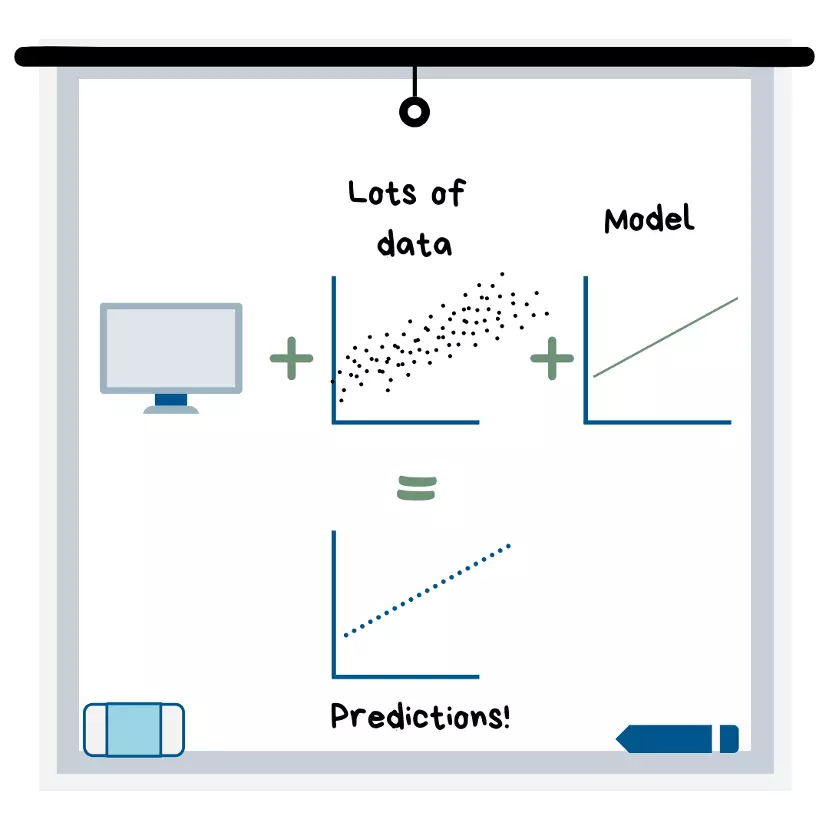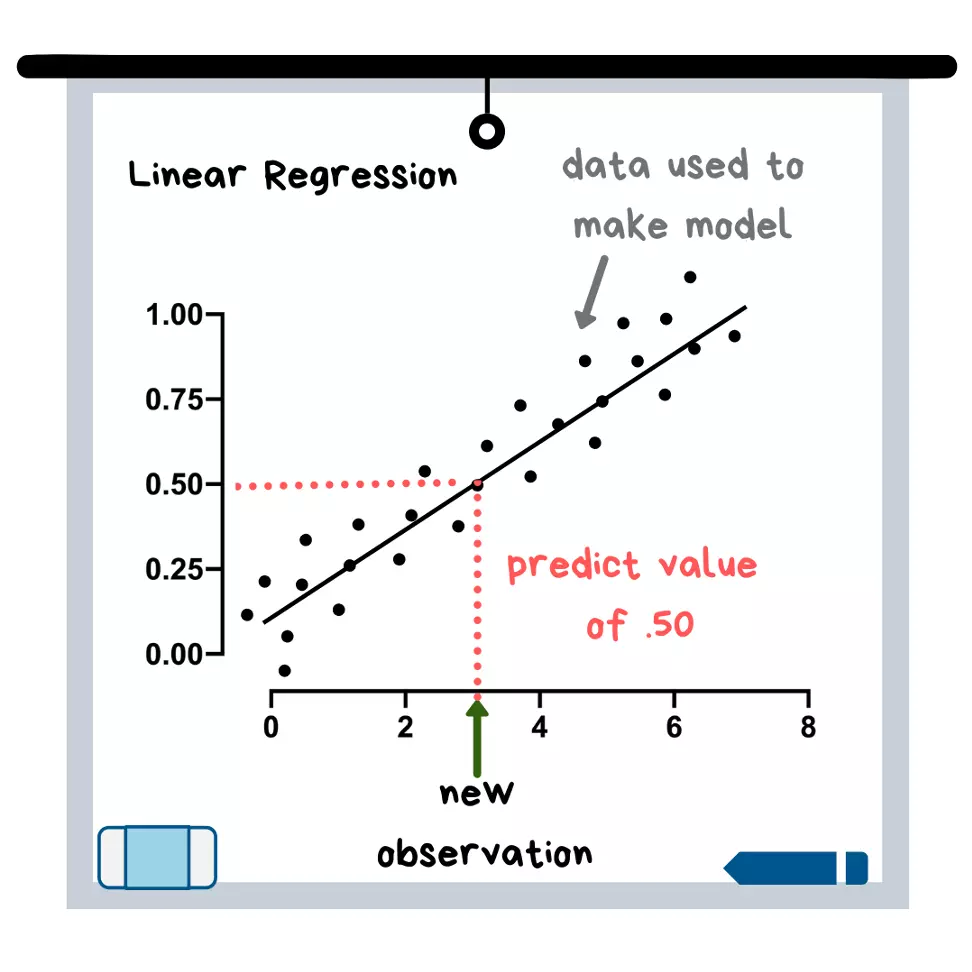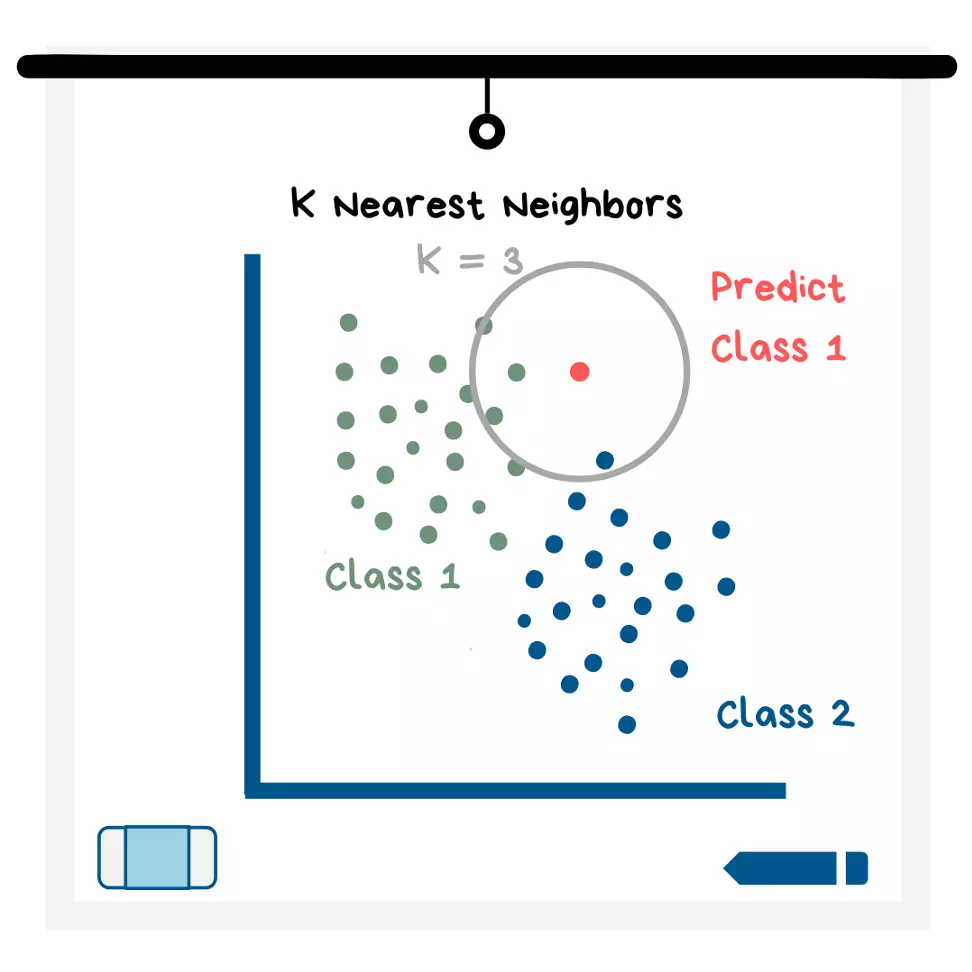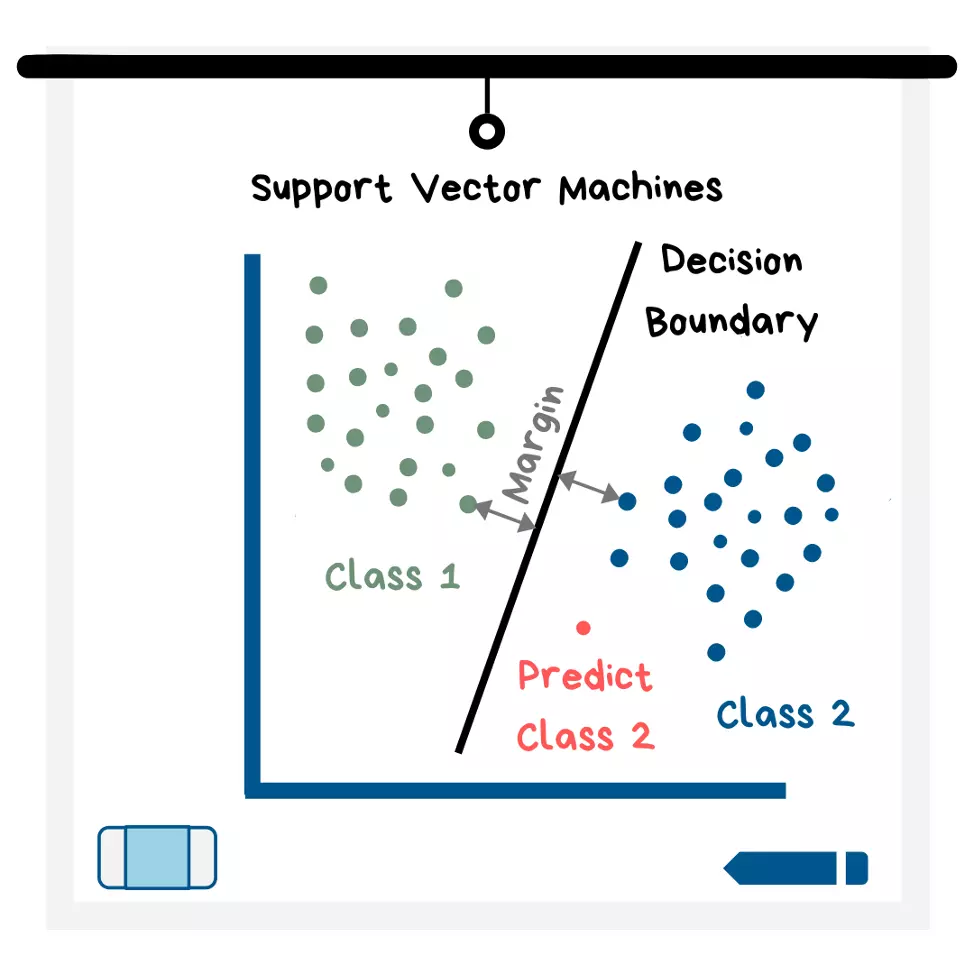# Machine learning models

Sociaro specialists have prepared a translation of another article that will help you understand what Machine Learning is.

Megan Dibble’s article from Medium Towards Data Science is the simplest explanation of how ML models work.
If you are new to data science, the title was not intended to offend you. This is my second post on a popular interview question that goes something like this: “explain to me [insert technical subject], as you would explain to a five year old.”

It turns out that reaching the level of understanding of a five-year-old child is quite difficult. So, while this article may not be entirely clear to a kindergartner, it will be clear to someone who has little to no experience in data science (and if you find that this is not the case, please write about it in the comments).

I will start by explaining the concept of machine learning as well as its different types and then move on to explaining simple models. I won’t go into the math too much, but I’m thinking about doing it in my future articles. Enjoy!## Definition of Machine Learning

Machine learning is when you load a large amount of data into a computer program and choose a model that will “fit” that data so that the computer (without your help) can come up with predictions. The computer builds models using algorithms that range from simple equations (such as the equation of a straight line) to very complex systems of logic/mathematics that allow the computer to make the best possible predictions.

The name machine learning is apt because once you choose a model to use and tune (in other words, improve with adjustments), the machine will use the model to learn patterns in your data. Then you can add new conditions (observations) and it will predict the result!

## Supervised machine learning definition

Supervised learning is a type of machine learning where the data you put into the model is “tagged”. A flag simply means that the result of the observation (i.e. the data series) is known. For example, if your model is trying to predict whether your friends will go golfing or not, you might have variables such as weather, day of the week, and so on. If your data is labelled, then your variable will have the value 1 if your friends went to play golf, and the value 0 if they didn’t.

## Unsupervised Machine Learning Definition

As you might have guessed, when it comes to labeled data, unsupervised learning is the opposite of supervised learning. In unsupervised learning, you can’t know if your friends are playing golf or not – only the computer can find patterns using the model to guess what has already happened or predict what will happen.

## Logistic regression

Logistic regression is used to solve a classification problem. This means that your target variable (the one you want to predict) is made up of categories. These categories can be yes/no, or something like a number from 1 to 10 that represents customer satisfaction. A logistic regression model uses an equation to create a curve on your data and then uses that curve to predict the results of a new observation.## Illustration of Logistic Regression

In the graph above, the new observation would have a prediction of 0 because it falls on the left side of the curve. If you look at the data that the curve is drawn from, this makes sense because in the “predicted value 0” area of ​​the graph, most of the points on the y-axis have a value of 0.

## Linear Regression

Quite often, linear regression becomes the first machine learning model that people learn. This is due to the fact that its algorithm (in other words, an equation) is quite simple to understand using only one variable x – you simply draw the most suitable line – a concept that is taught in elementary school. The best fitting line is then used to predict new data points (see illustration).

## Illustration of Linear Regression

Linear Regression is somewhat similar to logistic regression, but is used when the target variable is continuous, which means that it can take on almost any numerical value. In fact, any model with a continuous target variable can be classified as a “regression”. An example of a continuous variable would be the selling price of a house.Linear regression is well interpreted. The model equation contains coefficients for each variable, and these coefficients show how much the target variable changes with the slightest change in the independent variable (x-variable). If you show this with the selling prices of a house as an example, it means that you could look at the regression equation and say something like “oh, this tells me that for every additional 1m2 of the size of the house (x-variable), the selling price (target variable) is increased by \$25.”

## K Nearest Neighbors (KNN)

This model can be used for classification or for regression. The name – “To the Nearest Neighbors” should not confuse you. To begin with, the model displays all the data on a graph. The “K” part of the name refers to the number of nearest neighbor data points that the model looks at to determine what the predicted value should be (see illustration below). As a future data scientist, you choose a K value and you can play around with it to see which value makes the best predictions.Illustration of K Nearest Neighbors

All data points in the K=__ circle get a “vote” on what the value of the target variable should be for this new data point. The value that receives the most votes is the value that KNN predicts for the new data point. In the illustration above, 2 nearest neighbors are class 1 while 1 neighbors are class 2. So the model would predict class 1 for this data point.If the model predicts a numeric value and not a category, then all “votes” are numeric values , which are averaged to get the prediction.

## Support Vector Machines (SVMs)

The way support vectors work is that they establish boundaries between data points where the majority of one class falls on one side of the boundary (for example, in 2D it would be a line) and most of the other class falls on the other side of the boundary.

## Illustration of Support Vector Machines

The way it works is also that the machine seeks to find the boundary with the largest limit. The limit is determined by the distance between the boundary and the closest points of each class (see illustration). New data points are then built and placed in a specific class, depending on which side of the boundary they fall on.

I explain this model with a classification example, but you can also use it for regression!

Decision Trees & Random Forests

We are now ready to move on to unsupervised machine learning. Let me remind you that this means that our dataset is not labeled, so we do not know the results of our observations.

## K Means Clustering

When you use K clustering, you must start by assuming that there are K clusters in your dataset. Because you don’t know how many groups your data actually has, you should try different K values ​​and use visualization and metrics to figure out which K value is appropriate. The K means method works best with circular clusters of the same size.

This algorithm first selects the best K data points to form the center of each K cluster. Then, it repeats the following 2 steps for each point:

1. Assigns a data point to the closest cluster center

2. Creates a new center by taking the average of all data points from this cluster

## DBSCAN Clustering

The DBSCAN clustering model differs from the K means method in that you don’t need to enter a K value, and it can find clusters of any shape (see illustration below). Instead of specifying the number of clusters, you enter the minimum number of data points you want to be present in the cluster and the radius around the data point to find the cluster. DBSCAN will find the clusters for you! Further, you can change the values ​​used to create the model until you get clusters suitable for your dataset.In addition, the DBSCAN model classifies “noise” points (ie points that are far away from all other observations) for you. This model performs better than the K means method when the data points are very close together.

## Neural networks

In my opinion, neural networks are the coolest and most mysterious models. They are called neural networks because they are modeled after the neurons in our brain. These models work to find patterns in a dataset, sometimes they find patterns that a human could never find.

Neural networks work with more complex data, such as pictures or audio. They are behind a lot of the software features we see all the time these days, from facial recognition to text classification. Neural networks can be used when the data is labeled (i.e. supervised learning) as well as when the data is unlabeled (unsupervised learning)..

## Conclusion

I hope that this article has not only increased your understanding of the above models, but also helped you understand how cool and useful they are! When we let the computer do the work/learn, we can sit back and watch what patterns it finds. Sometimes it can all be confusing, because even experts don’t understand the exact logic by which a computer comes to a certain conclusion, but in some cases, all we care about is the quality of the forecast!

However, there are cases where we care about how the computer came up with a prediction, such as when we use a model to determine which job applicants are worthy of the first round of an interview. If you want to know more about this, here’s a TED talk that you can watch and appreciate even if you’re not a data scientist.